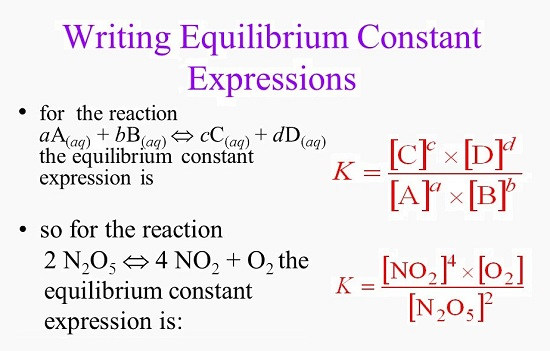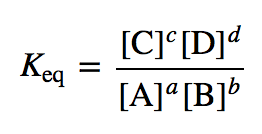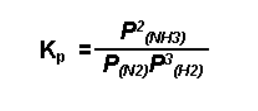# Equilibrium Expressions Study Guide

INTRODUCTION

The universe is always maintaining a state of equilibrium. If the equilibrium gets unbalanced, the energy flow of the universe gets disrupted. In the same way, nature also maintains a balance. The chemical equation for the various laws of physics also tells us about the maintenance of equilibrium. So, in chemistry, let us learn about the equilibrium constant expressions and how they will help us solve the various chemical reactions!

### WHAT ARE EQUILIBRIUM CONSTANTS?

In a chemical reaction, the equilibrium constant can be expressed as the ratio between the amount of reactant and the amount of product that is utilized to define the chemical behavior. The equilibrium constant is denoted by K, and it provides a mediator in the relation between a reactant and a product in chemical reactions.

For example, the equilibrium constant of concentration which is denoted by Kc, is the ratio between the product and the reactants of the chemical reaction.

Hence, in a chemical reaction,

The rate of forward reaction = the rate of the backward or reverse reaction

For example,

kf = kr

Or, kf × α × [A]ᵃ [B]ᵇ = kr × α × [C]ᶜ [D]ᵈ

The ratio of the rate constant of forward reaction to the rate constant of a backward reaction is constant and is called an equilibrium constant (Kequ). The rate constants are constants at a particular temperature.Source

### HOW TO WRITE EQUILIBRIUM EXPRESSIONS?

To write the equilibrium expression for a thermodynamic system in a state of equilibrium, you need to know:

• The balanced equation for the reaction
• The different phases, i.e., solid, liquid, gas, or dissolved, of each species involved in the reaction

### EQUILIBRIUM CONSTANT FORMULASource

Kequ = kf/kb = [X]ˣ [Y]ʸ / [L]ˡ [M]ᵐ = K꜀

Where

K꜀ = indicates the equilibrium constant measured in moles per liter.

For reactions involving gases: The equilibrium constant formula, in terms of partial pressure will be:

Kequ = kf/kb = [[pC]c [pD]d]/[[pA]a [pB]b] = Kp

Where

Kp indicates the equilibrium constant formula in terms of particle pressure.

• Larger Kc/Kp values denote higher product formation and higher percentage conversion.
• Medium Kc/Kp values reveal optimum product formation.
• Lower Kc/Kp values denote lower product formation and lower percentage conversion.

### FAQs

1. How do you create an equilibrium equation?

If you have an equation like the decomposition of N₂O4 to NO₂, the forward and backward reactions for the system contain a single elementary reaction. Hence the forward and reverse equation can be written as:

Forward rate = kf [N₂O₄]

Reverse rate = kr [NO₂]²

So, in equilibrium, we know that the forward rate = the reverse rate,

``                     kf [N₂O₄] = kr [NO₂]²``

It can be written as:

``                    kf / kr =  [N₂O₄] / [NO₂]²``

2. How do you write equilibrium expressions KP?

When more than one of the elements or compounds in a thermodynamic system exists in the gaseous phase, the partial pressure of that element or compound can be used in the equilibrium expression. Dissolved elements are still expressed as moles per liter (molarity). For example:Source

3. How do you write an equilibrium constant?

The equilibrium constant is represented by the letter K, and the equilibrium constant is the relative concentrations of reactants and products at equilibrium.

We hope you enjoyed studying this lesson and learned something cool about Equilibrium Expressions! Join our Discord community to get any questions you may have answered and to engage with other students just like you! Don’t forget to download our App to experience our fun VR classrooms – we promise it makes studying much more fun! 😎

## SOURCES

1. The Equilibrium Constant: https://chem.libretexts.org/Bookshelves/General_Chemistry/Map%3A_General_Chemistry_(Petrucci_et_al.)/15%3A_Principles_of_Chemical_Equilibrium/15.2%3A_The_Equilibrium_Constant_Expression. Accessed 7th March 2022.
2. Writing Equilibrium Expressions: https://www.chem.purdue.edu/gchelp/howtosolveit/Equilibrium/Writing_Equilibrium_Expressions.htm#Kconcentration.Accessed 7th March 2022.
]]>